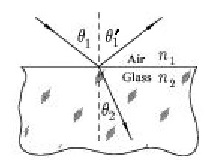# Problem: True or False. The light ray that makes the angle θ1' is the incident ray.

###### FREE Expert Solution

Snell's law:

$\overline{){{\mathbf{\eta }}}_{{\mathbf{1}}}{\mathbf{s}}{\mathbf{i}}{\mathbf{n}}{{\mathbf{\theta }}}_{{\mathbf{1}}}{\mathbf{=}}{{\mathbf{\eta }}}_{{\mathbf{2}}}{\mathbf{s}}{\mathbf{i}}{\mathbf{n}}{{\mathbf{\theta }}}_{{\mathbf{2}}}}$

The incident light upon striking a surface is split into the reflected ray and a transmitted ray.

The angle between the reflected ray and the normal and the angle between the incident ray and the normal are equal according to the the law of reflection.###### Problem Details

True or False. The light ray that makes the angle θ1' is the incident ray.